# Graphing Linear Equations Linear Equation An equation for

• Slides: 31Graphing Linear Equations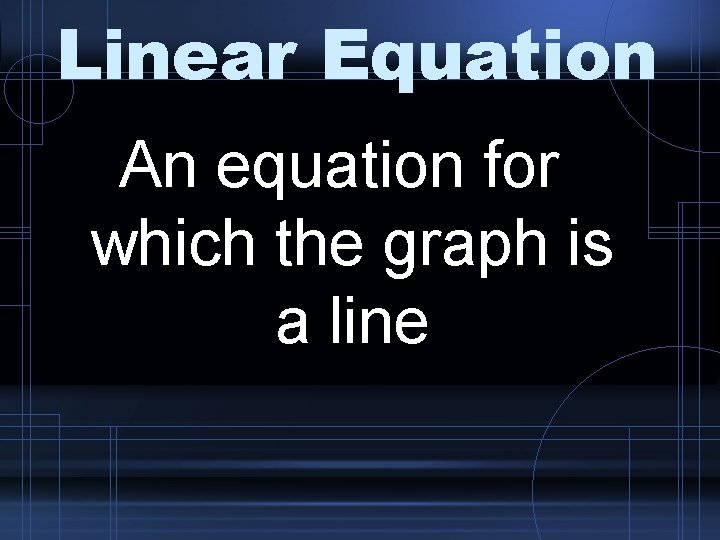Linear Equation An equation for which the graph is a line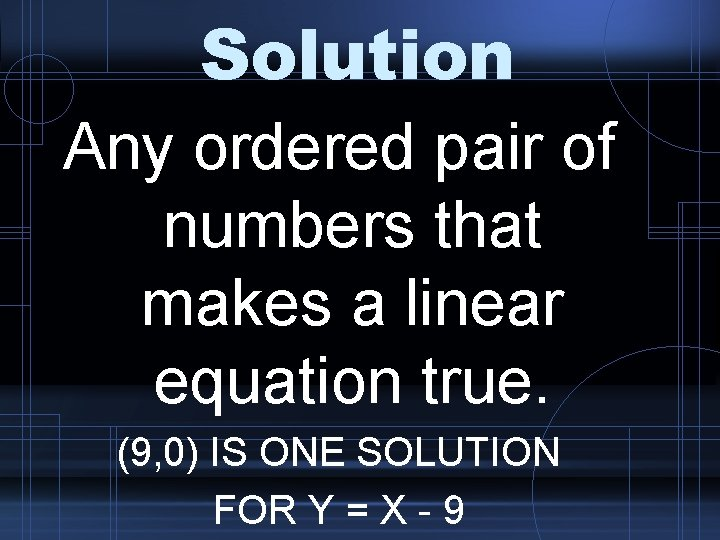Solution Any ordered pair of numbers that makes a linear equation true. (9, 0) IS ONE SOLUTION FOR Y = X - 9Linear Equation Example: y=x+3Graphing Step 1: ~ Three Point Method ~ Choose 3 values for x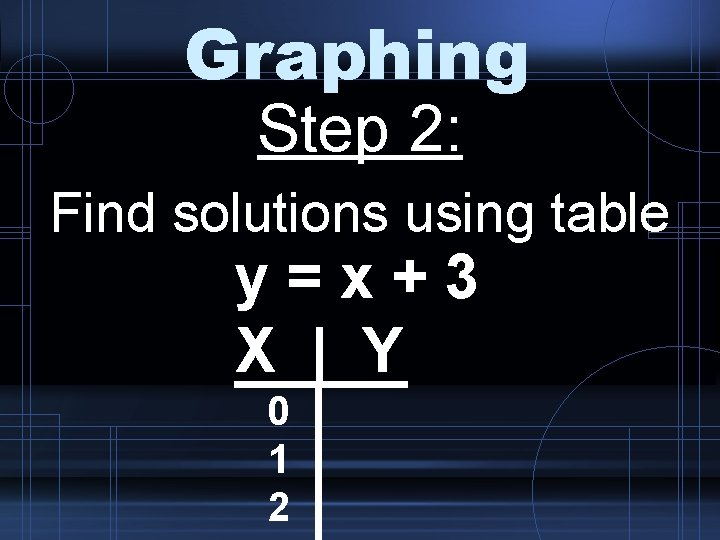Graphing Step 2: Find solutions using table y=x+3 X | Y 0 1 2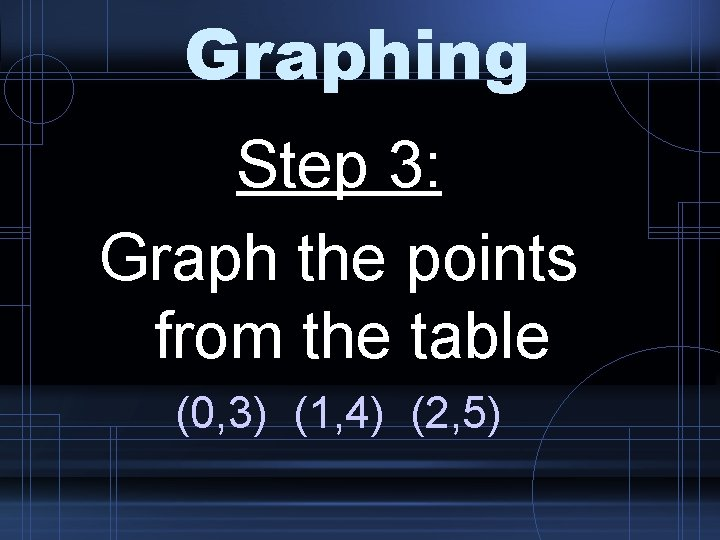Graphing Step 3: Graph the points from the table (0, 3) (1, 4) (2, 5)Graphing Step 4: Draw a line to connect themTry These • Graph using a table (3 point method) 1) y = x + 3 2) y = x - 4Slope-Intercept y = mx + b m = slope b = y-interceptSlope-Intercept • The m in the slope-intercept form is ALWAYS attached to the X variable. • The slope is NEVER including the X variable • For example, if given y = 3 x + 4 the slope is 3 NOT 3 x.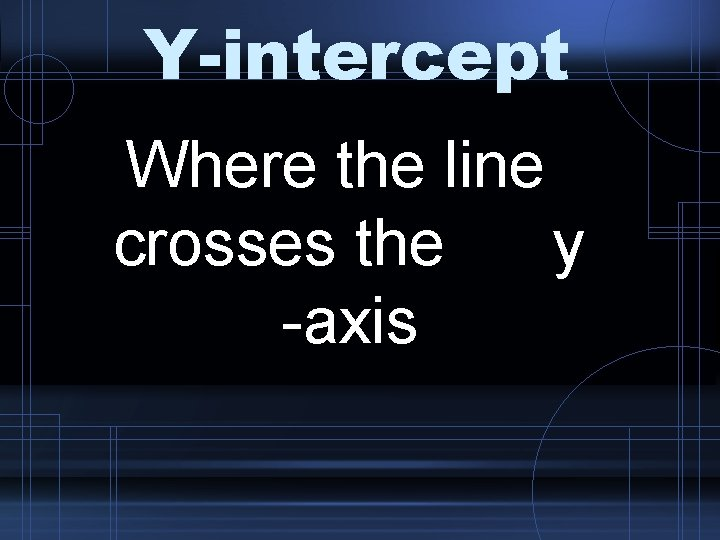Y-intercept Where the line crosses the y -axisY-intercept The y-intercept has an x-coordinate of ZERO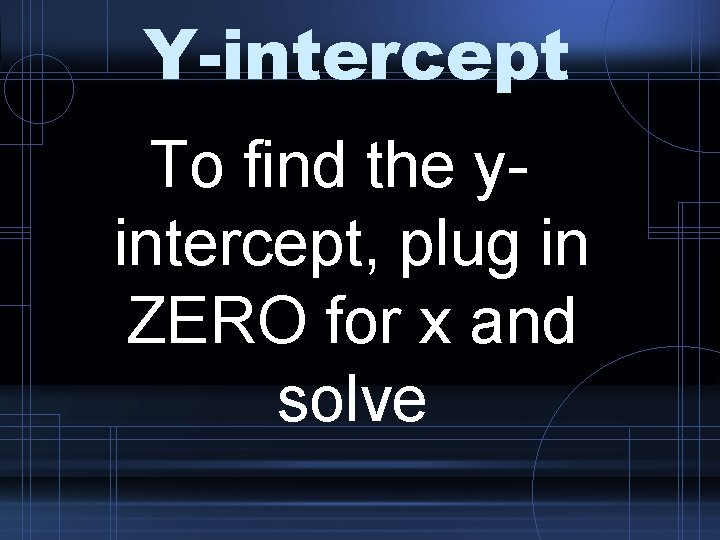Y-intercept To find the yintercept, plug in ZERO for x and solve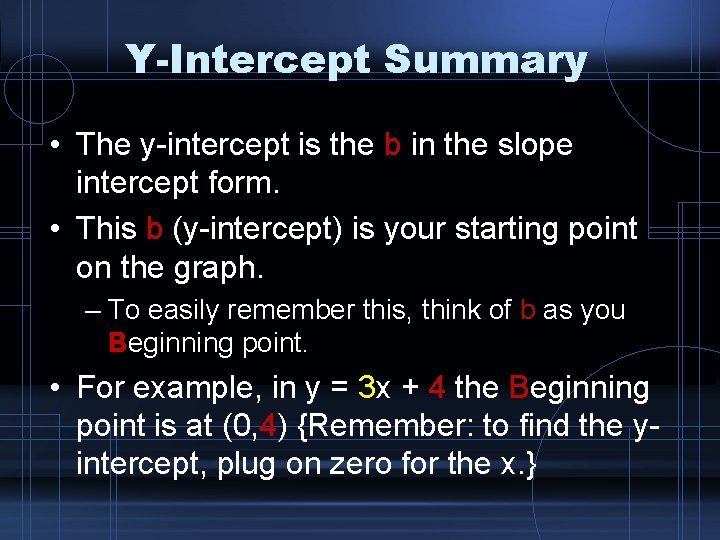Y-Intercept Summary • The y-intercept is the b in the slope intercept form. • This b (y-intercept) is your starting point on the graph. – To easily remember this, think of b as you Beginning point. • For example, in y = 3 x + 4 the Beginning point is at (0, 4) {Remember: to find the yintercept, plug on zero for the x. }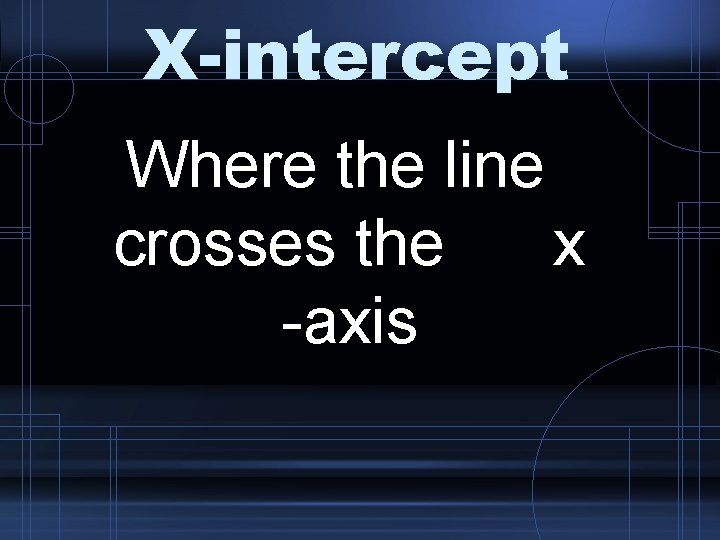X-intercept Where the line crosses the x -axis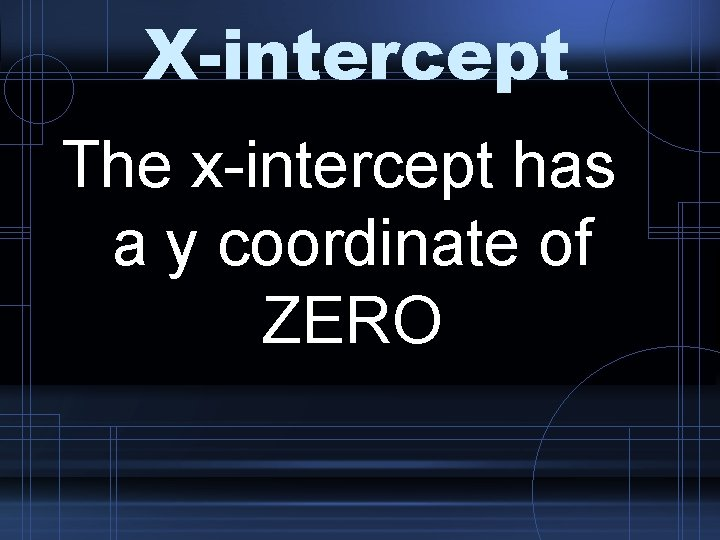X-intercept The x-intercept has a y coordinate of ZERO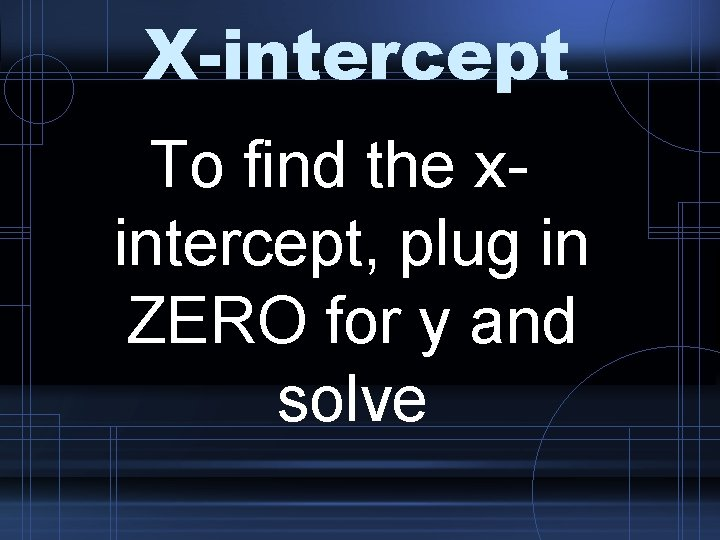X-intercept To find the xintercept, plug in ZERO for y and solve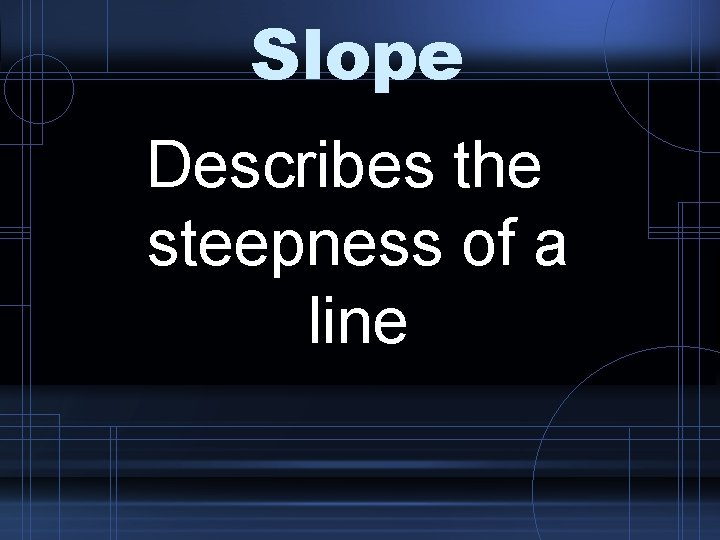Slope Describes the steepness of a lineSlope Equal to: Rise Run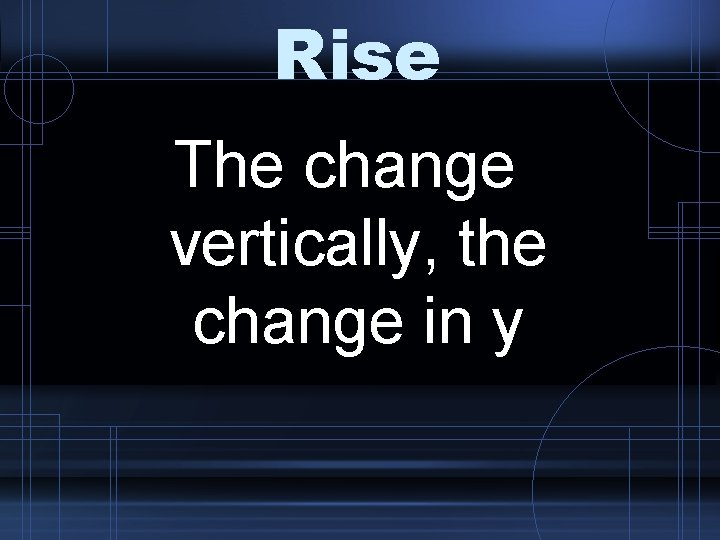Rise The change vertically, the change in y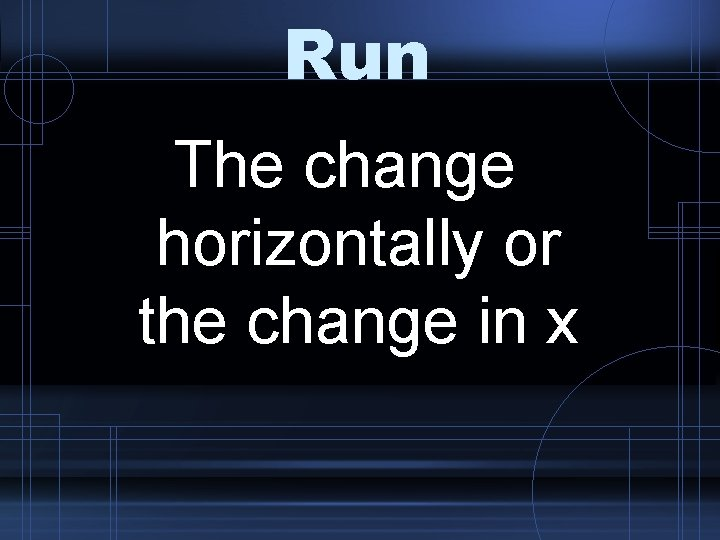Run The change horizontally or the change in xFinding Slope Step 1: Find 2 points on a line (2, 3) (5, 4) (x , y ) 1 1 2 2Finding Slope Step 2: Find the RISE between these 2 points Y-Y = 2 1 4 -3=1Finding Slope Step 3: Find the RUN between these 2 points X-X = 2 1 5 -2=3Finding Slope Step 4: Write the RISE over RUN as a ratio Y-Y 2 1 X-X 2 1 = 1 3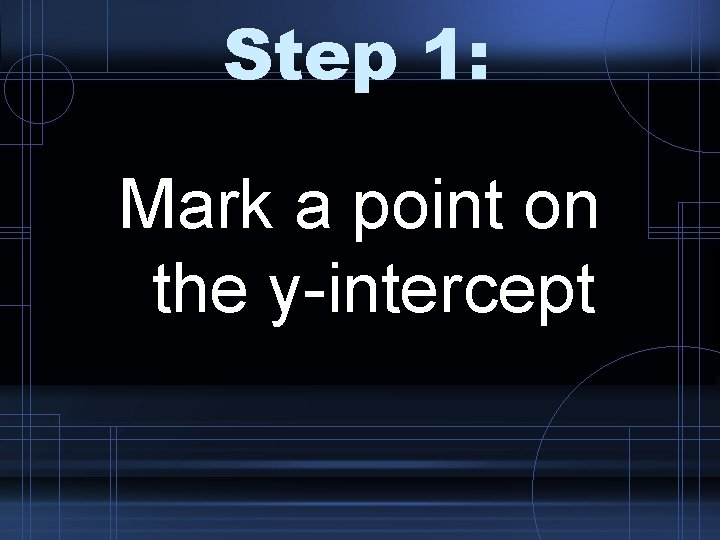Step 1: Mark a point on the y-intercept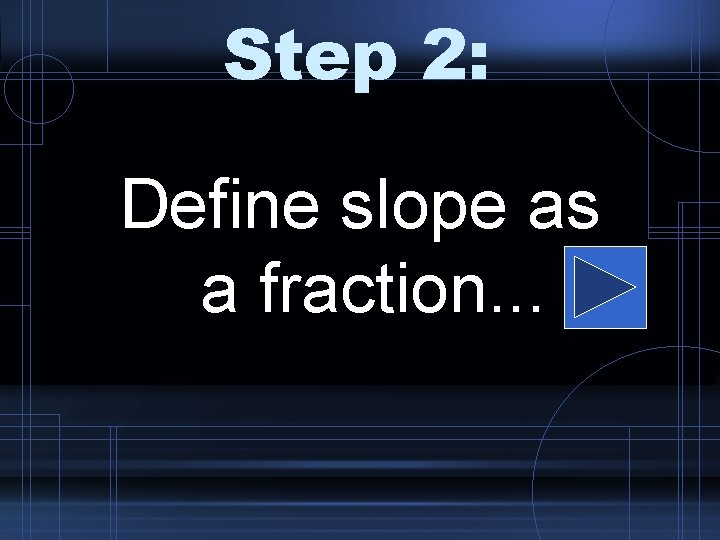Step 2: Define slope as a fraction. . .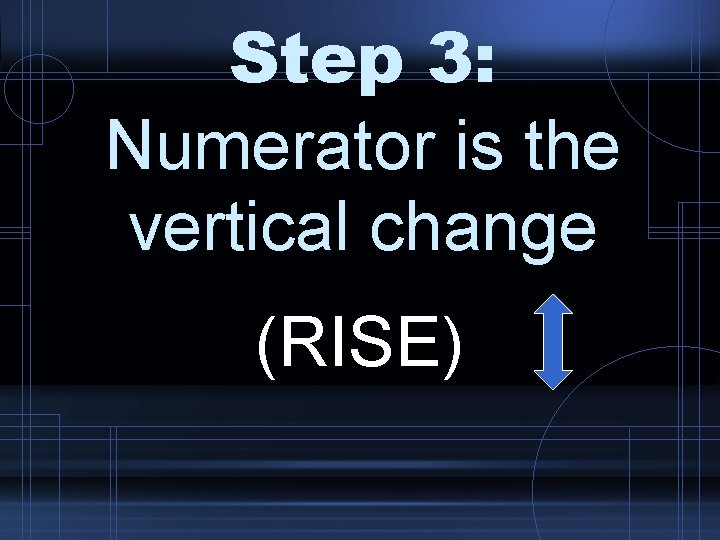Step 3: Numerator is the vertical change (RISE)Step 4: Denominator is the horizontal change (RUN)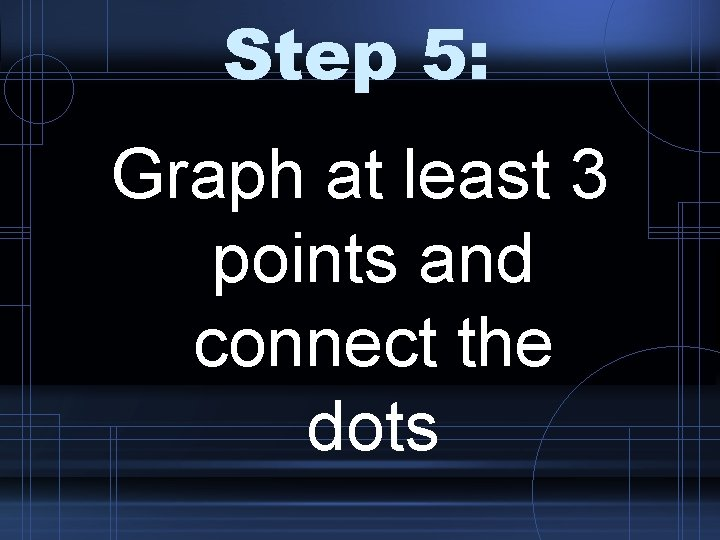Step 5: Graph at least 3 points and connect the dots